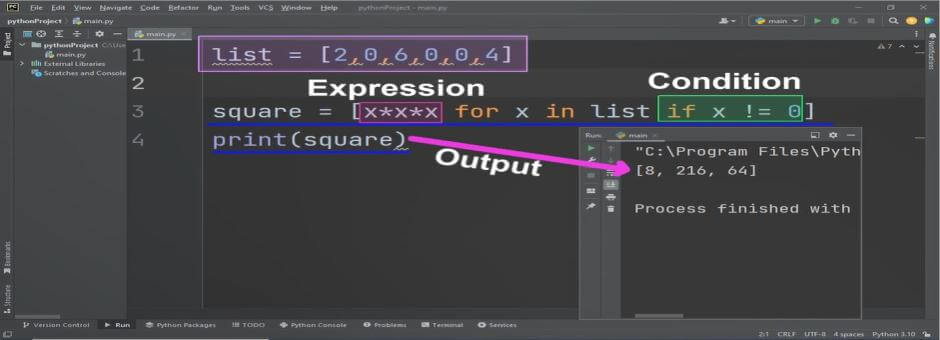# Python List Comprehension

Python list Comprehension is the compact and efficient method for creating new list from existing list with same or modified data. This feature is uniquely available in python programming language. List Comprehension use an expression for the assignment of new data items in list. This technique also used for itterative structure to traverse data items of the provided list. This iteration return one after another value/data item from the list and that become part of new python list.

We can also use some relational expression to filter existing list data items using if statement.

## List Comprehension Syntax

list Comprehension syntax used an expression, for loop and condition (optional).

``list = [expression/variable for variable in list if condition == True] ``

Here start of list Comprehension is using expression/variable, this variable is the return from iterative statement. Secondly in for loop we have to use list data structure such as existing python list. And in the last there may be an expression that must return TRUE to return particular value for storing in new list.

## Python list comprehension if

We can also use list comprehension with if statement, In this case any valid relational or logical expression is used. For loop will return only those values to new list which satisfies the provided expression as TRUE.

### Example

oldlist = list[1,2,3,4,5,6,7,8,9,10]

newlist = [x for x in oldlist if x > 5]

print(newlist)

The result of above example will be a new list containing only numbers greater than 5 such as.

#### Output:

``[6, 7, 8, 9]``

## List Comprehension Expression

We can also use python range function directly in list comprehension. Such as in next example we age going to store squares of numbers from 1 to 10.

### Example

square = [ x * x for x in range(1,11)]
print(square)

#### Output

``[1, 4, 9, 16, 25, 36, 49, 64, 81]``

## Uses of List Comprehension

We can use list comprehension to save our programming time. The source will be more compact and more readable with the use of list comprehension. Many developers use this in more advance and complicated manners. Where more complicated expressions and conditions/relational expressions are used.

## Example List Comprehension

In this example we will use the existing list of numbers, and use List Comprehension for creating a new list having the cubes of existing list values. We also use an if condition to check either data item is greater than 0. This will calculate cube of those data values which are greater than zero and store in the new list.

list = [2,0,6,0,0,4]

square = [x*x*x for x in list if x != 0]
print(square)

### Output:

The output of above python code will be as.